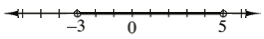### Home > INT3 > Chapter 3 > Lesson 3.2.4 > Problem3-111

3-111.

Solve each inequality and graph the solution on a number line.

1. $x^2-2x-15<0$

See the homework help for part (b) of problem 3-103.

$−31. $| 3 x - 2 | \geq 10$

Change the inequality to an absolute value equation and solve it to determine the boundary points.

Use the boundary points to create regions on a number line and then test each region to determine if the region is “true” or “false.”
Refer to the Math Notes box in Lesson 3.2.2 for additional help.